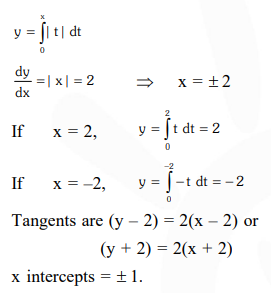# Solve this following`
Question:

The intercepts on $x$-axis made by tangents to the curve, $y=\int_{0}^{x}|t| d t, x \in R$, which are parallel to the line $\mathrm{y}=2 \mathrm{x}$, are equal to

1. $\pm 1$

2. $\pm 2$

3. $\pm 3$

4. $\pm 4$

Correct Option: 1

Solution: